# NCERT Solutions For Class 10 Maths Chapter 3 Exercise 3.5 – Pair of Linear Equations in two Variables

Download NCERT Solutions For Class 10 Maths Chapter 3 Exercise 3.5 – Pair of Linear Equations in two Variables. This Exercise contains 4 questions, for which detailed answers have been provided in this note. In case you are looking at studying the remaining Exercise for Class 10 for Maths NCERT solutions for other Chapters, you can click the link at the end of this Note.

### NCERT Solutions For Class 10 Maths Chapter 3 Exercise 3.5 – Pair of Linear Equations in two Variables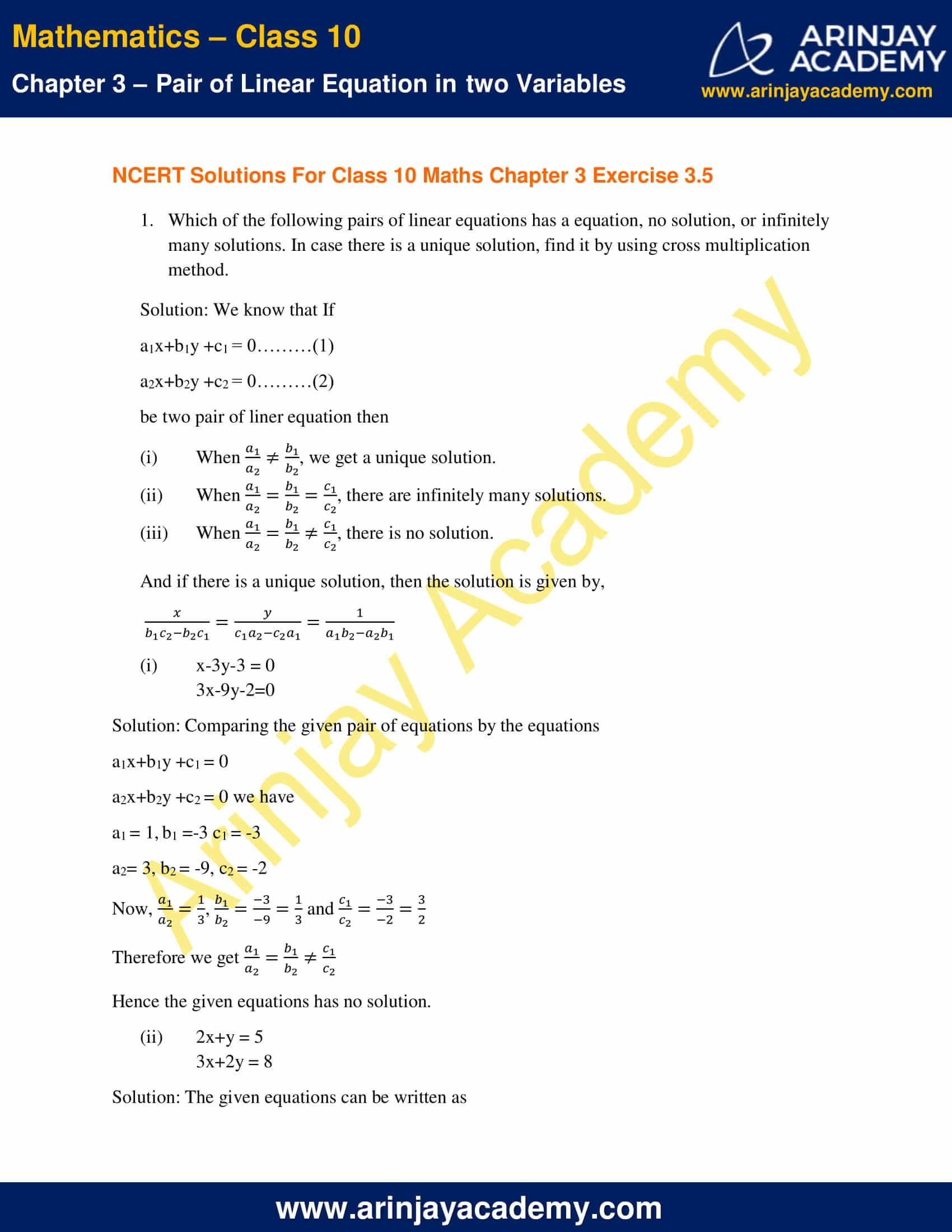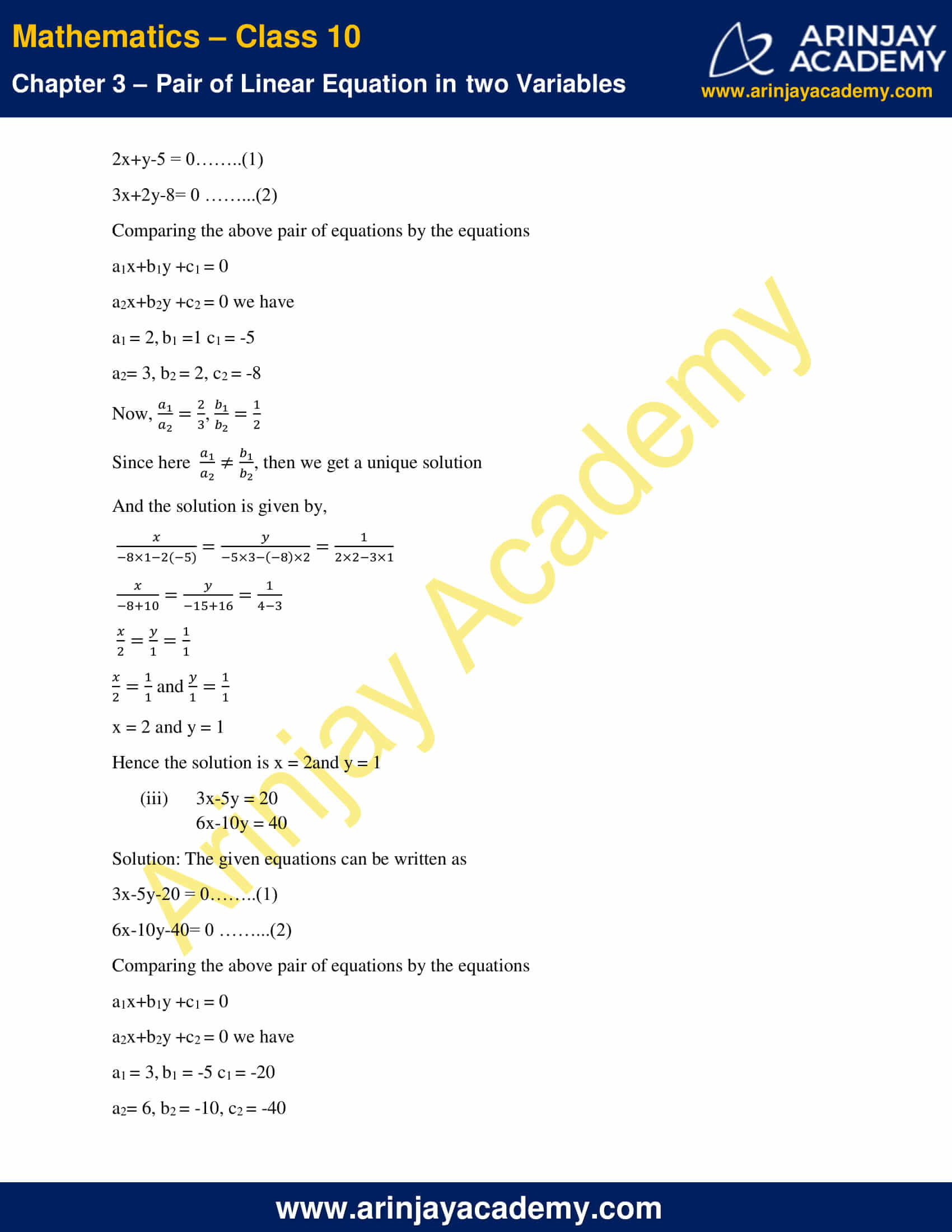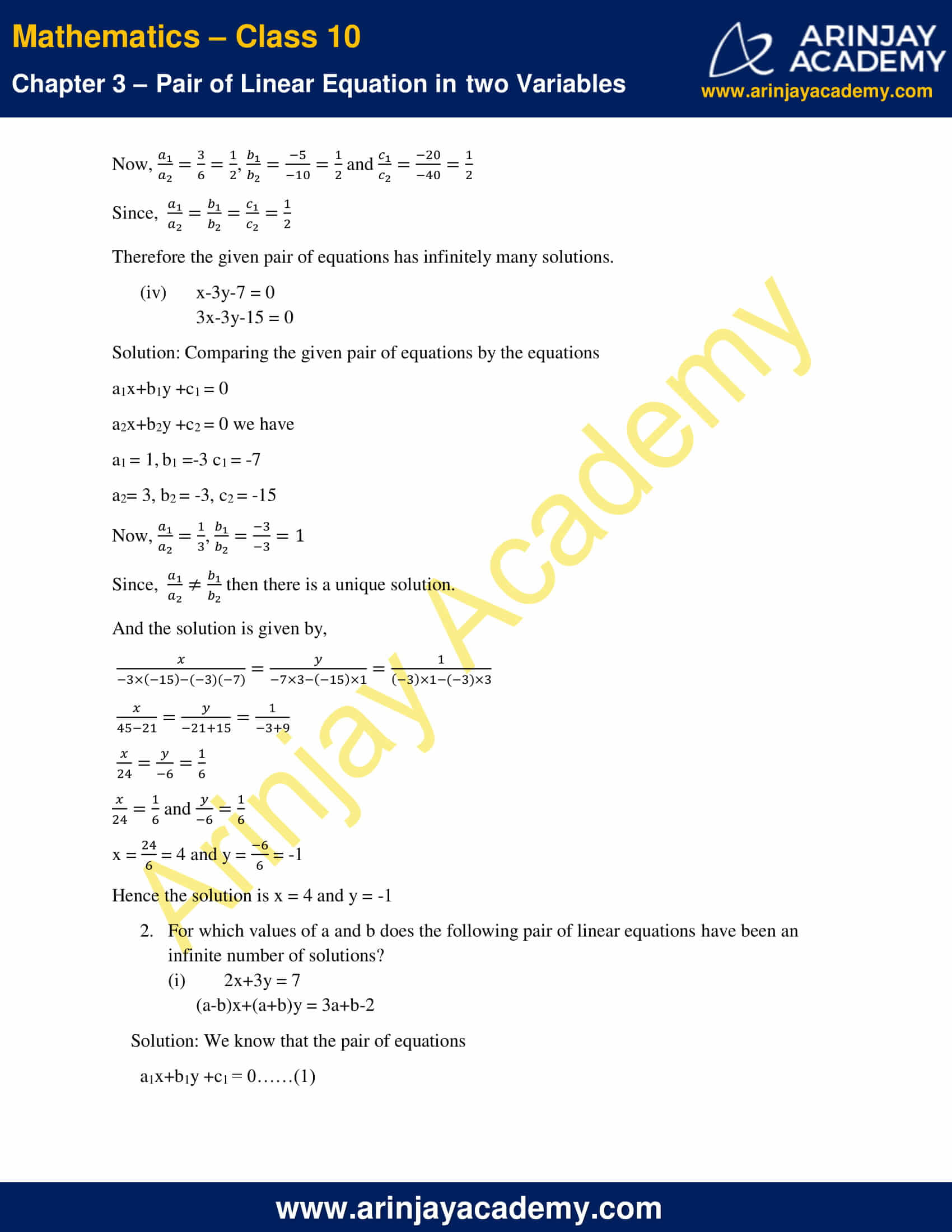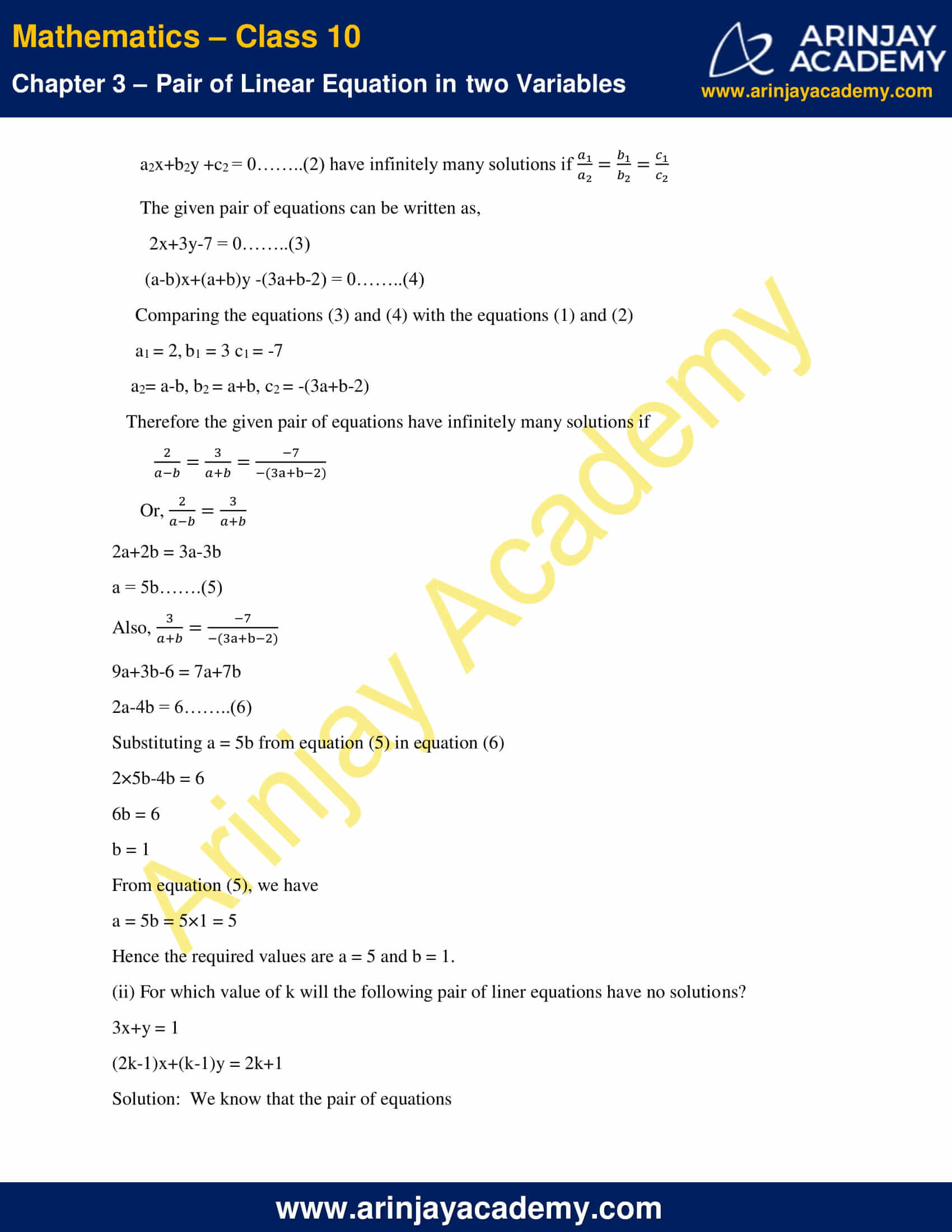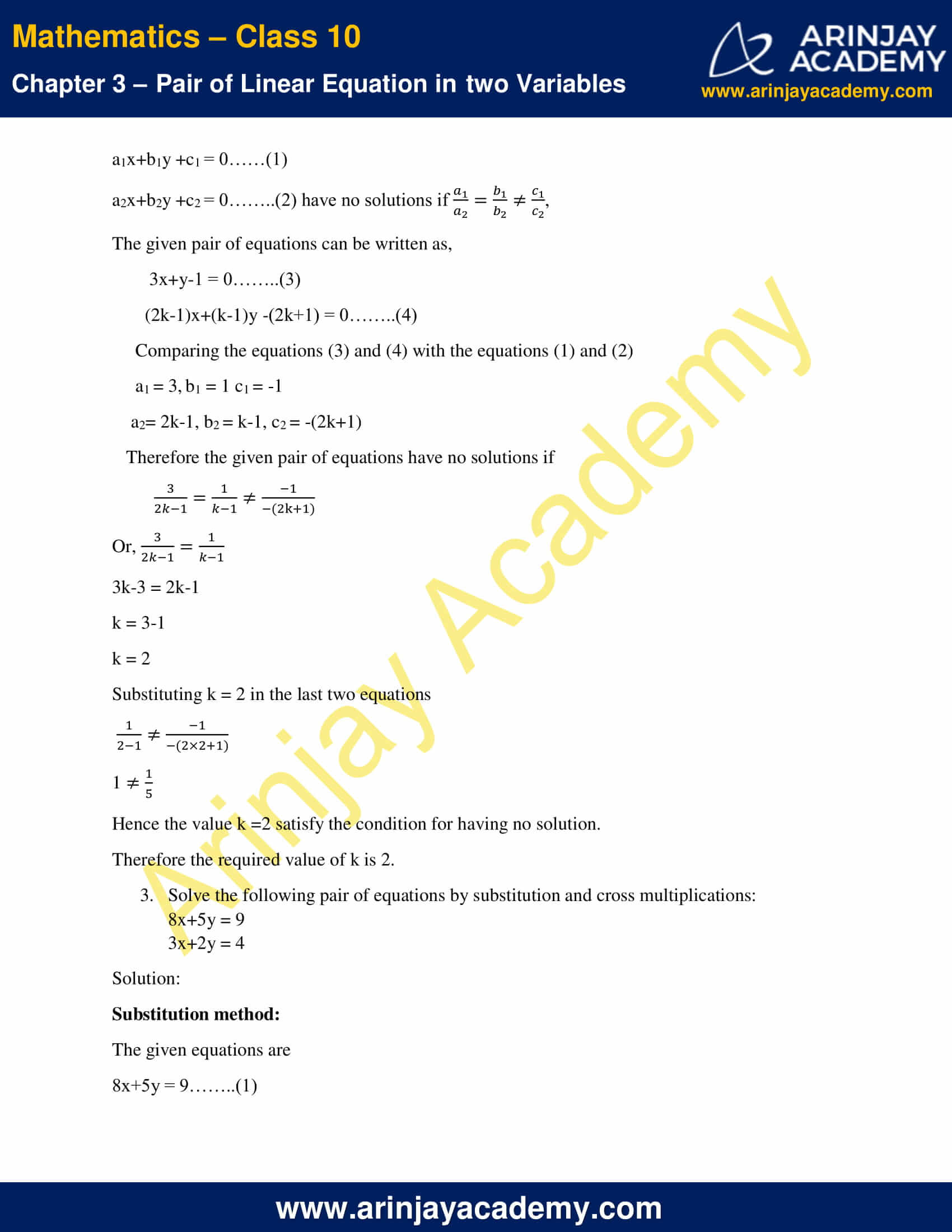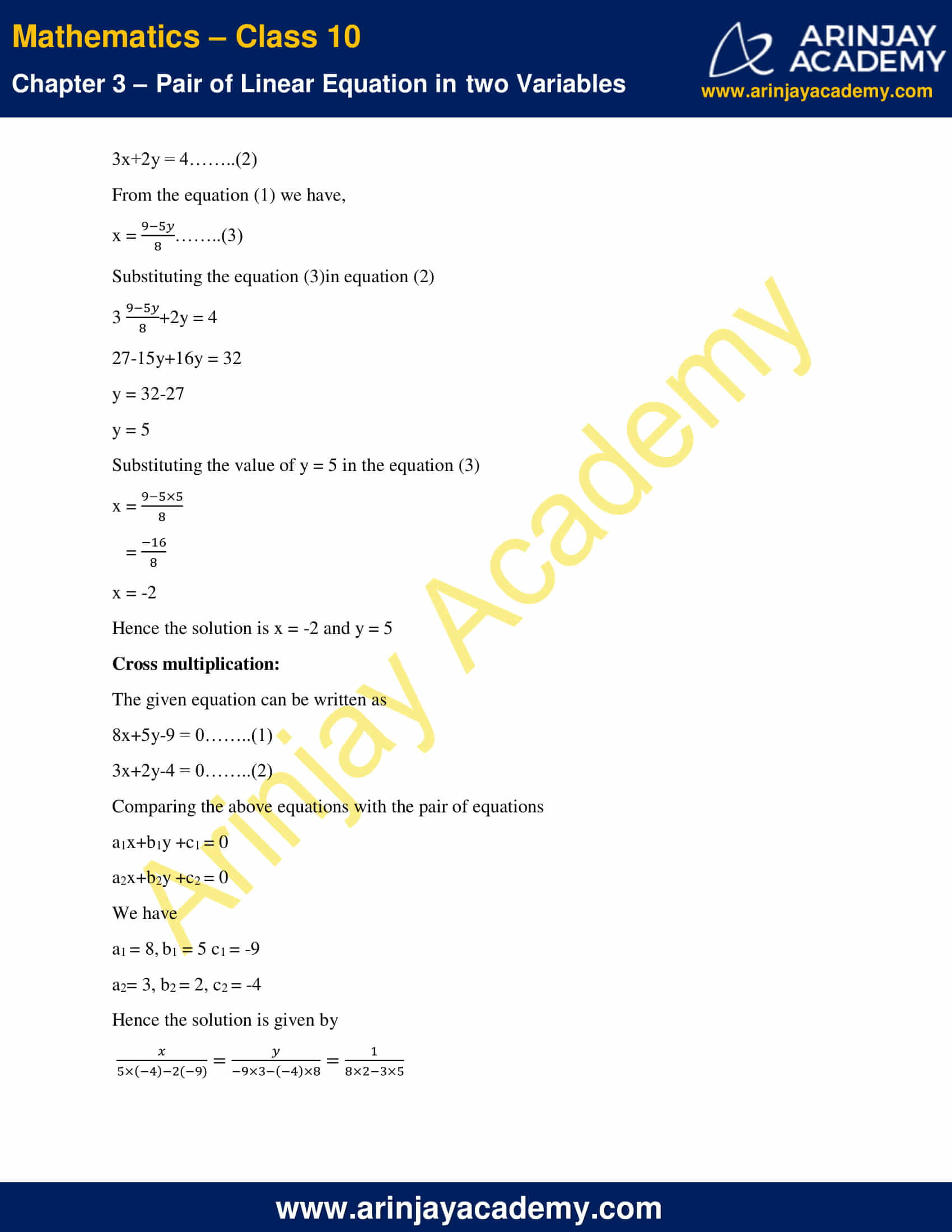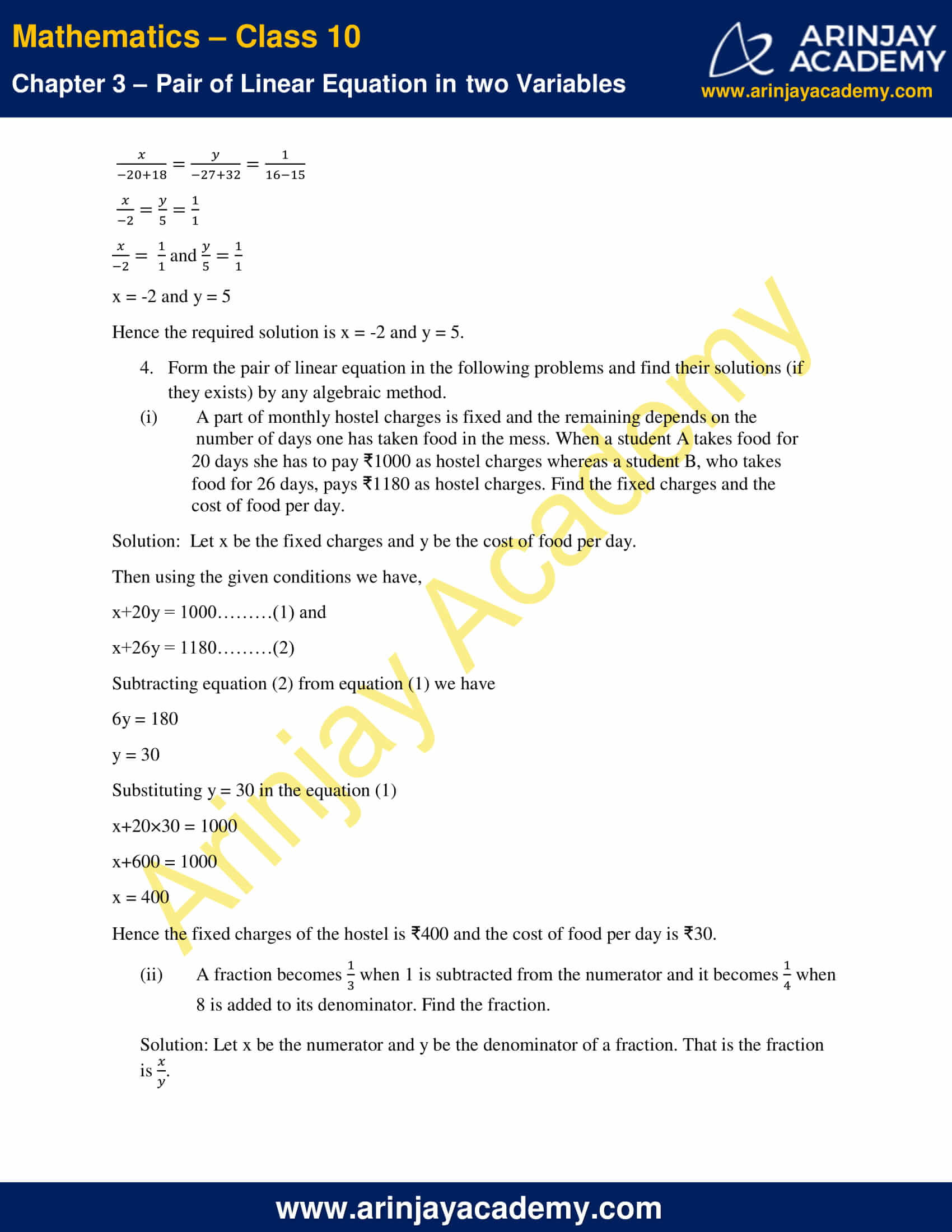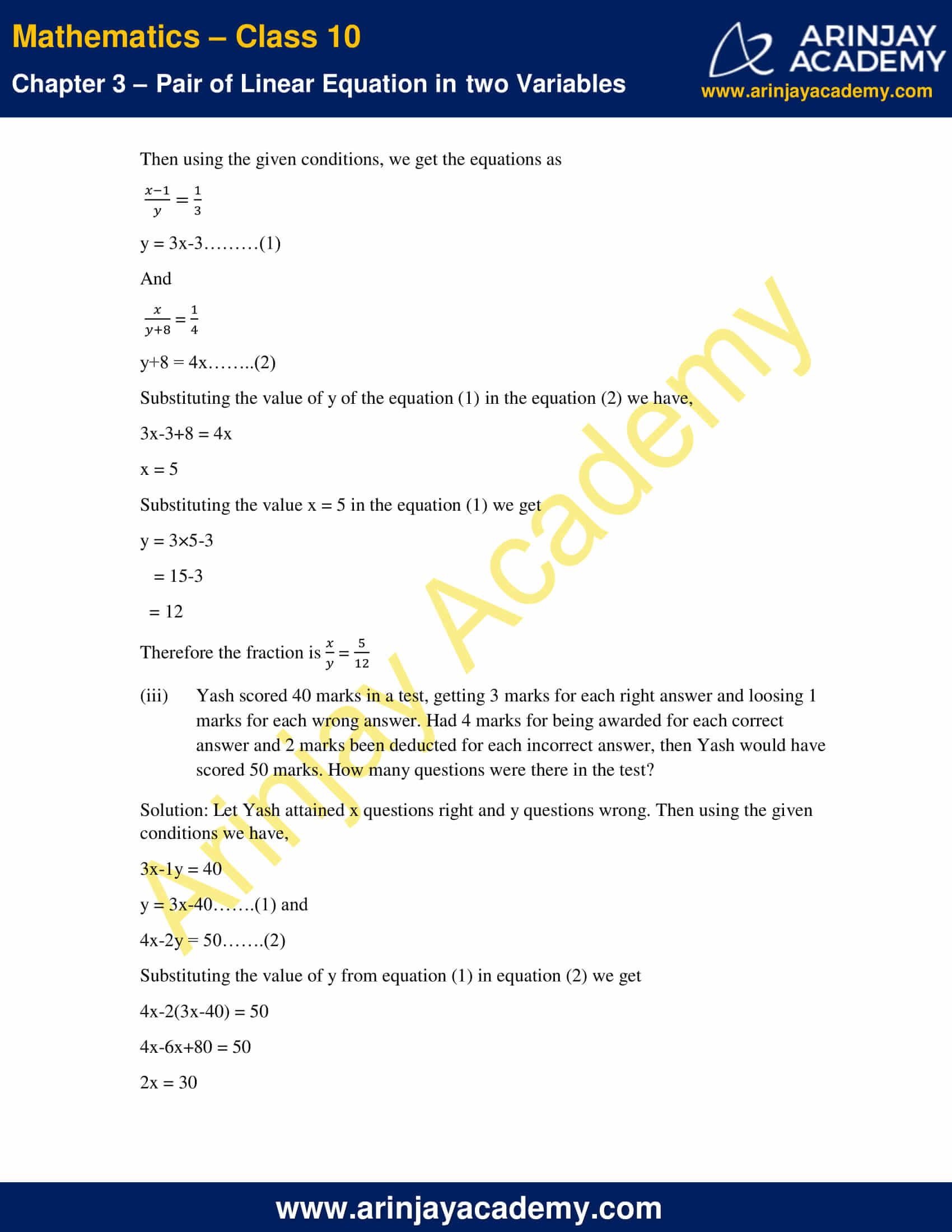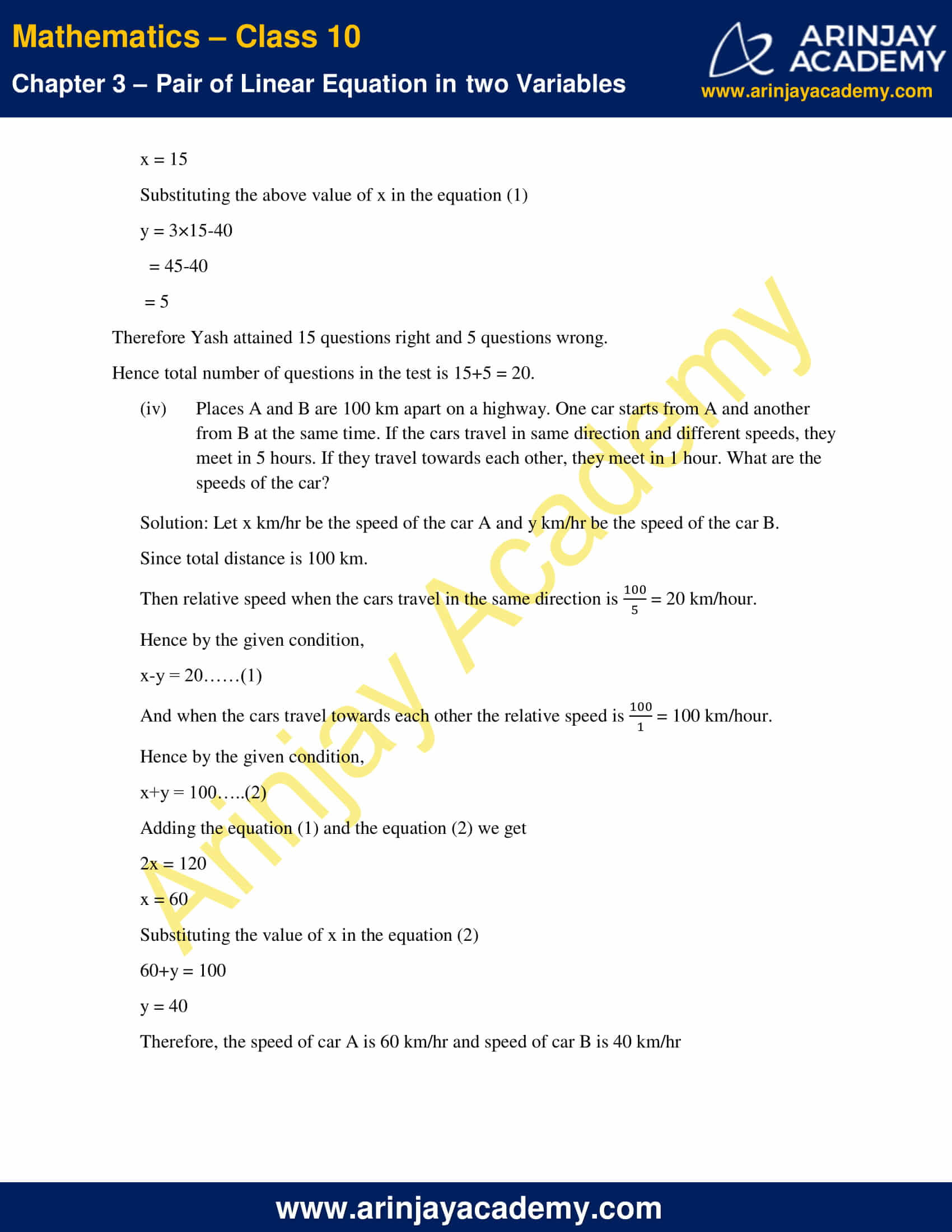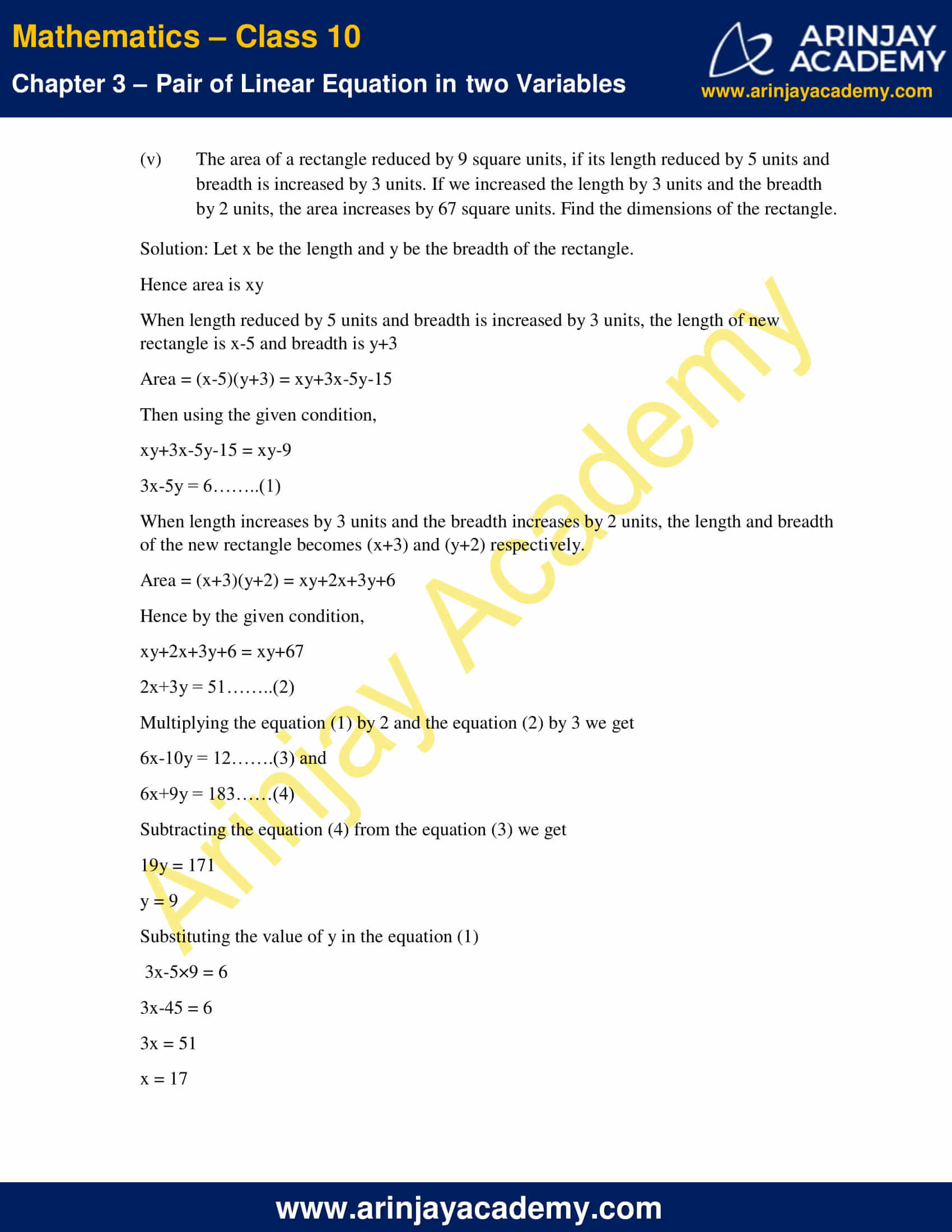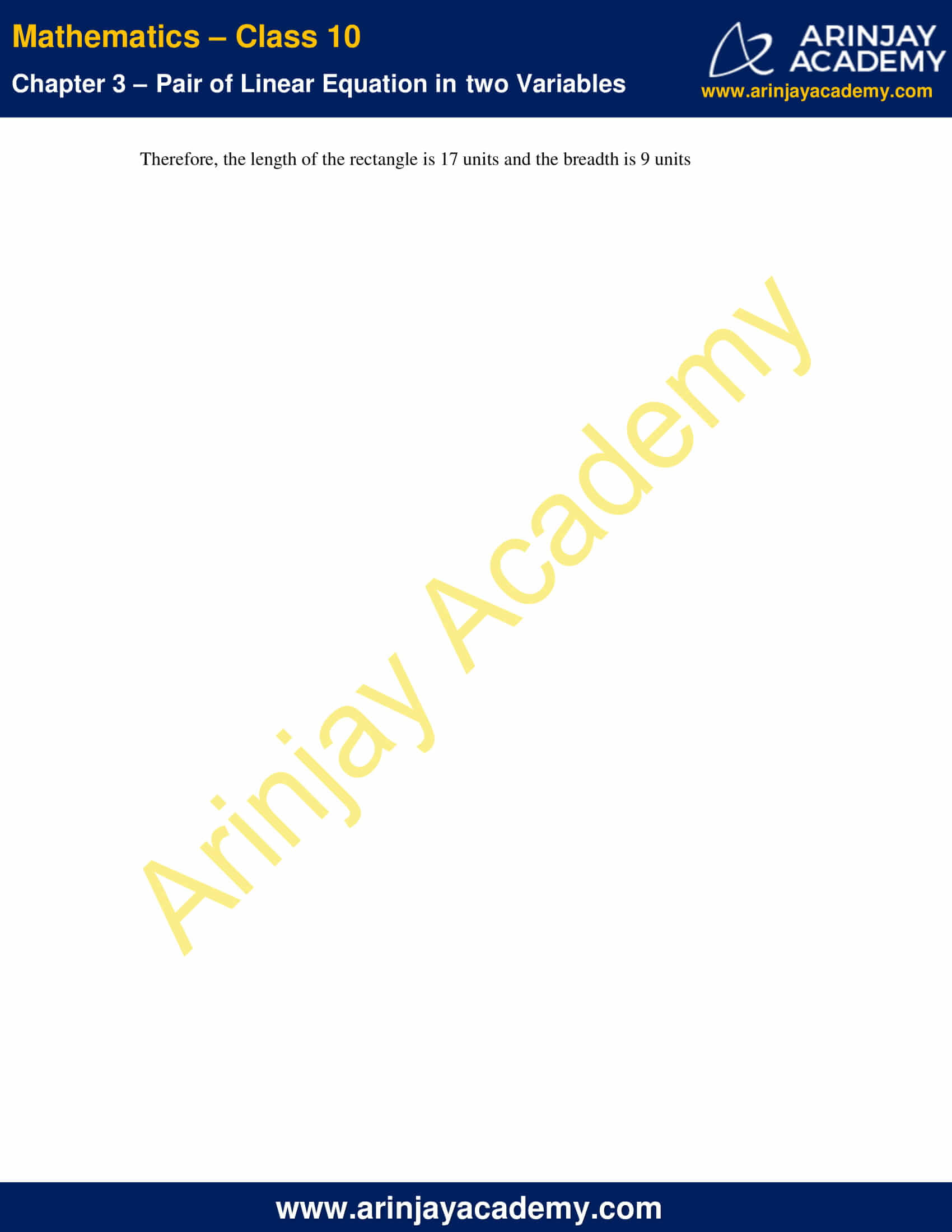NCERT Solutions For Class 10 Maths Chapter 3 Exercise 3.5 – Pair of Linear Equations in two Variables

1. Which of the following pairs of linear equations has a equation, no solution, or infinitely many solutions. In case there is a unique solution, find it by using cross multiplication method.

Solution: We know that If

a1x+b1y +c1 = 0………(1)

a2x+b2y +c2 = 0………(2)

be two pair of liner equation then

When$\cfrac { { a }_{ 1 } }{ { a }_{ 2 } } \neq \cfrac { { b }_{ 1 } }{ { b }_{ 2 } }$, we get a unique solution.

When$\cfrac { { a }_{ 1 } }{ { a }_{ 2 } } =\cfrac { { b }_{ 1 } }{ { b }_{ 2 } } =\cfrac { { c }_{ 1 } }{ { c }_{ 2 } }$, there are infinitely many solutions.

When$\cfrac { { a }_{ 1 } }{ { a }_{ 2 } } =\cfrac { { b }_{ 1 } }{ { b }_{ 2 } } \neq \cfrac { { c }_{ 1 } }{ { c }_{ 2 } }$, there is no solution.

And if there is a unique solution, then the solution is given by,$\cfrac { x }{ b_{ 1 }{ c }_{ 2 }\quad -\quad b_{ 2 }{ c }_{ 1 } } =\cfrac { y }{ c_{ 1 }{ a }_{ 2 }\quad -\quad c_{ 2 }{ a }_{ 1 } } =\cfrac { 1 }{ a_{ 1 }{ b }_{ 2 }\quad -\quad a_{ 2 }{ b }_{ 1 } }$

(i) x-3y-3 = 0

3x-9y-2=0

Solution: Comparing the given pair of equations by the equations

a1x+b1y +c1 = 0

a2x+b2y +c2 = 0 we have

a1 = 1, b1 =-3 c1 = -3

a2= 3, b2 = -9, c2 = -2

Now,$\cfrac { { a }_{ 1 } }{ { a }_{ 2 } } =\cfrac { 1 }{ 3 }$,$\cfrac { { b }_{ 1 } }{ { b }_{ 2 } } =\cfrac { -3 }{ -9 } =\cfrac { 1 }{ 3 }$,$\cfrac { { c }_{ 1 } }{ { c }_{ 2 } } =\cfrac { -3 }{ -2 } =\cfrac { 3 }{ 2 }$

Therefore we get$\cfrac { { a }_{ 1 } }{ { a }_{ 2 } } =\cfrac { { b }_{ 1 } }{ { b }_{ 2 } } \neq \cfrac { { c }_{ 1 } }{ { c }_{ 2 } }$

Hence the given equations has no solution.

(ii) 2x+y = 5

3x+2y = 8

Solution: The given equations can be written as

2x+y-5 = 0……..(1)

3x+2y-8= 0 ………(2)

Comparing the above pair of equations by the equations

a1x+b1y +c1 = 0

a2x+b2y +c2 = 0 we have

a1 = 2, b1 =1 c1 = -5

a2= 3, b2 = 2, c2 = -8

Now,$\cfrac { { a }_{ 1 } }{ { a }_{ 2 } } =\cfrac { 2 }{ 3 }$,$\cfrac { { b }_{ 1 } }{ { b }_{ 2 } } =\cfrac { 1 }{ 2 }$,

Since here$\cfrac { { a }_{ 1 } }{ { a }_{ 2 } } \neq \cfrac { { b }_{ 1 } }{ { b }_{ 2 } }$, then we get a unique solution

And the solution is given by,$\cfrac { x }{ -8\times 1\quad -\quad 2(-5) } =\cfrac { y }{ -5\times 3\quad -\quad (-8)\times 2 } =\cfrac { 1 }{ 2\times 2\quad -\quad 3\times 1 }$$\cfrac { x }{ -8+10 } =\cfrac { y }{ -15+16 } =\cfrac { 1 }{ 4-3 }$$\cfrac { x }{ 2 } =\cfrac { y }{ 1 } =\cfrac { 1 }{ 1 }$$\cfrac { x }{ 2 } =\cfrac { 1 }{ 1 }$ and$\cfrac { y }{ 1 } =\cfrac { 1 }{ 1 }$

x = 2 and y = 1

Hence the solution is x = 2and y = 1

(iii) 3x-5y = 20

6x-10y = 40

Solution: The given equations can be written as

3x-5y-20 = 0……..(1)

6x-10y-40= 0 ………(2)

Comparing the above pair of equations by the equations

a1x+b1y +c1 = 0

a2x+b2y +c2 = 0 we have

a1 = 3, b1 = -5 c1 = -20

a2= 6, b2 = -10, c2 = -40

Now,$\cfrac { { a }_{ 1 } }{ { a }_{ 2 } } =\cfrac { 3 }{ 6 } = \cfrac { 1 }{ 2 }$,$\cfrac { { b }_{ 1 } }{ { b }_{ 2 } } =\cfrac { -5 }{ -10 } =\cfrac { 1 }{ 2 }$,$\cfrac { { c }_{ 1 } }{ { c }_{ 2 } } =\cfrac { -20 }{ -40 } =\cfrac { 1 }{ 2 }$

Since,$\cfrac { { a }_{ 1 } }{ { a }_{ 2 } } =\cfrac { { b }_{ 1 } }{ { b }_{ 2 } } =\cfrac { { c }_{ 1 } }{ { c }_{ 2 } } =\cfrac { 1 }{ 2 }$

Therefore the given pair of equations has infinitely many solutions.

(iv) x-3y-7 = 0

3x-3y-15 = 0

Solution: Comparing the given pair of equations by the equations

a1x+b1y +c1 = 0

a2x+b2y +c2 = 0 we have

a1 = 1, b1 =-3 c1 = -7

a2= 3, b2 = -3, c2 = -15

Now,$\cfrac { { a }_{ 1 } }{ { a }_{ 2 } } =\cfrac { 1 }{ 3 }$,$\cfrac { { b }_{ 1 } }{ { b }_{ 2 } } =\cfrac { -3 }{ -3 }$ = 1

Since$\cfrac { { a }_{ 1 } }{ { a }_{ 2 } } \neq \cfrac { { b }_{ 1 } }{ { b }_{ 2 } }$, then there is a unique solution

And the solution is given by,$\cfrac { x }{ -3\times (-15)\quad -\quad (-3)(-7) } =\cfrac { y }{ -7\times 3\quad -\quad (-15)\times 1 } =\cfrac { 1 }{ (-3)\times 1\quad -\quad (-3)\times 3 }$$\cfrac { x }{ 45-21 } =\cfrac { y }{ -21+15 } =\cfrac { 1 }{ -3+9 }$$\cfrac { x }{ 24 } =\cfrac { y }{ -6 } =\cfrac { 1 }{ 6 }$$\cfrac { x }{ 24 } =\cfrac { 1 }{ 6 }$ and$\cfrac { y }{ -6 } =\cfrac { 1 }{ 6 }$

x=$\cfrac { 24 }{ 6 }$=4

y =$\cfrac { -6 }{ 6 }$=-1

Hence the solution is x = 4 and y = -1

2. For which values of a and b does the following pair of linear equations have been an infinite number of solutions?

(i) 2x+3y = 7

(a-b)x+(a+b)y = 3a+b-2

Solution: We know that the pair of equations

a1x+b1y +c1 = 0……(1)

a2x+b2y +c2 = 0……..(2) have infinitely many solutions if$\cfrac { { a }_{ 1 } }{ { a }_{ 2 } } =\cfrac { { b }_{ 1 } }{ { b }_{ 2 } } =\cfrac { { c }_{ 1 } }{ { c }_{ 2 } }$

The given pair of equations can be written as,

2x+3y-7 = 0……..(3)

(a-b)x+(a+b)y -(3a+b-2) = 0……..(4)

Comparing the equations (3) and (4) with the equations (1) and (2)

a1 = 2, b1 = 3 c1 = -7

a2= a-b, b2 = a+b, c2 = -(3a+b-2)

Therefore the given pair of equations have infinitely many solutions if$\cfrac { 2 }{ { a }-b } =\cfrac { 3 }{ a+b } =\cfrac { -7 }{ -(3a+b-2) }$

or,$\cfrac { 2 }{ { a }-b } =\cfrac { 3 }{ a+b }$

2a+2b = 3a-3b

a = 5b…….(5)

Also,$\cfrac { 3 }{ a+b } =\cfrac { -7 }{ -(3a+b-2) }$

9a+3b-6 = 7a+7b

2a-4b = 6……..(6)

Substituting a = 5b from equation (5) in equation (6)

2×5b-4b = 6

6b = 6

b = 1

From equation (5), we have

a = 5b = 5×1 = 5

Hence the required values are a = 5 and b = 1.

(ii) For which value of k will the following pair of liner equations have no solutions?

3x+y = 1

(2k-1)x+(k-1)y = 2k+1

Solution:  We know that the pair of equations

a1x+b1y +c1 = 0……(1)

a2x+b2y +c2 = 0……..(2) have no solutions if$\cfrac { { a }_{ 1 } }{ { a }_{ 2 } } =\cfrac { { b }_{ 1 } }{ { b }_{ 2 } } \neq \cfrac { { c }_{ 1 } }{ { c }_{ 2 } }$,

The given pair of equations can be written as,

3x+y-1 = 0……..(3)

(2k-1)x+(k-1)y -(2k+1) = 0……..(4)

Comparing the equations (3) and (4) with the equations (1) and (2)

a1 = 3, b1 = 1 c1 = -1

a2= 2k-1, b2 = k-1, c2 = -(2k+1)

Therefore the given pair of equations have no solutions if$\cfrac { 3 }{ 2k-1 } =\cfrac { 1 }{ k-1 } \neq \cfrac { -1 }{ -(2k+1) }$

or,$\cfrac { 3 }{ 2k-1 } =\cfrac { 1 }{ k-1 }$

3k-3 = 2k-1

k = 3-1

k = 2

Substituting k = 2 in the last two equations$\cfrac { 1 }{ 2-1 } \neq \cfrac { -1 }{ -(2\times 2+1) }$$1\quad \neq \cfrac { 1 }{ 5 }$

Hence the value k =2 satisfy the condition for having no solution.

Therefore the required value of k is 2.

3. Solve the following pair of equations by substitution and cross multiplications:

8x+5y = 9

3x+2y = 4

Solution:

Substitution method:

The given equations are

8x+5y = 9……..(1)

3x+2y = 4……..(2)

From the equation (1) we have,

x =$\cfrac { 9-5y }{ 8 }$……..(3)

Substituting the equation (3)in equation (2)$3\times \cfrac { 9-5y }{ 8 }$ + 2y = 4

27-15y+16y = 32

y = 32-27

y = 5

Substituting the value of y = 5 in the equation (3)$x\quad =\quad \cfrac { 9-5\times 5 }{ 8 }$$x\quad =\quad \cfrac { -16 }{ 8 }$

x = -2

Hence the solution is x = -2 and y = 5

Cross multiplication:

The given equation can be written as

8x+5y-9 = 0……..(1)

3x+2y-4 = 0……..(2)

Comparing the above equations with the pair of equations

a1x+b1y +c1 = 0

a2x+b2y +c2 = 0

We have

a1 = 8, b1 = 5 c1 = -9

a2= 3, b2 = 2, c2 = -4

Hence the solution is given by$\cfrac { x }{ 5\times (-4)\quad -\quad 2(-9) } =\cfrac { y }{ -9\times 3\quad -\quad (-4)\times 8 } =\cfrac { 1 }{ 8\times 2\quad -\quad 3\times 5 }$$\cfrac { x }{ -20+18 } =\cfrac { y }{ -27+32 } =\cfrac { 1 }{ 16-15 }$$\cfrac { x }{ -2 } =\cfrac { y }{ 5 } =\cfrac { 1 }{ 1 }$$\cfrac { x }{ -2 } =\cfrac { 1 }{ 1 }$ and$\cfrac { y }{ 5 } =\cfrac { 1 }{ 1 }$

x = -2 and y = 5

Hence the required solution is x = -2 and y = 5.

4. Form the pair of linear equation in the following problems and find their solutions (if they exists) by any algebraic method.

(i) A part of monthly hostel charges is fixed and the remaining depends on the number of days one has taken food in the mess. When a student A takes food for 20 days she has to pay ₹1000 as hostel charges whereas a student B, who takes food for 26 days, pays ₹1180 as hostel charges. Find the fixed charges and the cost of food per day.

Solution:  Let x be the fixed charges and y be the cost of food per day.

Then using the given conditions we have,

x+20y = 1000………(1) and

x+26y = 1180………(2)

Subtracting equation (2) from equation (1) we have

6y = 180

y = 30

Substituting y = 30 in the equation (1)

x+20×30 = 1000

x+600 = 1000

x = 400

Hence the fixed charges of the hostel is ₹400 and the cost of food per day is ₹30.

(ii) A fraction becomes$\cfrac { 1 }{ 3 }$ when 1 is subtracted from the numerator and it becomes$\cfrac { 1 }{ 4 }$ when 8 is added to its denominator. Find the fraction.

Solution: Let x be the numerator and y be the denominator of a fraction. That is the fraction is$\cfrac { x }{ y }$.

Then using the given conditions, we get the equations as$\cfrac { x-1 }{ y }$ =$\cfrac { 1 }{ 3 }$

y = 3x-3………(1)

And$\cfrac { x }{ y+8 }$  =$\cfrac { 1 }{ 4 }$

y+8 = 4x……..(2)

Substituting the value of y of the equation (1) in the equation (2) we have,

3x-3+8 = 4x

x = 5

Substituting the value x = 5 in the equation (1) we get

y = 3×5-3

= 15-3

= 12

Therefore the fraction is$\cfrac { x }{ y }$ =$\cfrac { 5 }{ 12 }$

(iii) Yash scored 40 marks in a test, getting 3 marks for each right answer and loosing 1 marks for each wrong answer. Had 4 marks for being awarded for each correct answer and 2 marks been deducted for each incorrect answer, then Yash would have scored 50 marks. How many questions were there in the test?

Solution: Let Yash attained x questions right and y questions wrong. Then using the given conditions we have,

3x-1y = 40

y = 3x-40…….(1) and

4x-2y = 50…….(2)

Substituting the value of y from equation (1) in equation (2) we get

4x-2(3x-40) = 50

4x-6x+80 = 50

2x = 30

x = 15

Substituting the above value of x in the equation (1)

y = 3×15-40

= 45-40

= 5

Therefore Yash attained 15 questions right and 5 questions wrong.

Hence total number of questions in the test is 15+5 = 20.

(iv) Places A and B are 100 km apart on a highway. One car starts from A and another from B at the same time. If the cars travel in same direction and different speeds, they meet in 5 hours. If they travel towards each other, they meet in 1 hour. What are the speeds of the car?

Solution: Let x km/hr be the speed of the car A and y km/hr be the speed of the car B.

Since total distance is 100 km.

Then relative speed when the cars travel in the same direction is$\cfrac { 100 }{ 5 }$ = 20 km/hour.

Hence by the given condition,

x-y = 20……(1)

And when the cars travel towards each other the relative speed is$\cfrac { 100 }{ 1 }$ = 100 km/hour.

Hence by the given condition,

x+y = 100…..(2)

Adding the equation (1) and the equation (2) we get

2x = 120

x = 60

Substituting the value of x in the equation (2)

60+y = 100

y = 40

Therefore, the speed of car A is 60 km/hr and speed of car B is 40 km/hr

(v) The area of a rectangle reduced by 9 square units, if its length reduced by 5 units and breadth is increased by 3 units. If we increased the length by 3 units and the breadth by 2 units, the area increases by 67 square units. Find the dimensions of the rectangle.

Solution: Let x be the length and y be the breadth of the rectangle.

Hence area is xy

When length reduced by 5 units and breadth is increased by 3 units, the length of new rectangle is x-5 and breadth is y+3

Area = (x-5)(y+3) = xy+3x-5y-15

Then using the given condition,

xy+3x-5y-15 = xy-9

3x-5y = 6……..(1)

When length increases by 3 units and the breadth increases by 2 units, the length and breadth of the new rectangle becomes (x+3) and (y+2) respectively.

Area = (x+3)(y+2) = xy+2x+3y+6

Hence by the given condition,

xy+2x+3y+6 = xy+67

2x+3y = 51……..(2)

Multiplying the equation (1) by 2 and the equation (2) by 3 we get

6x-10y = 12…….(3) and

6x+9y = 183……(4)

Subtracting the equation (4) from the equation (3) we get

19y = 171

y = 9

Substituting the value of y in the equation (1)

3x-5×9 = 6

3x-45 = 6

3x = 51

x = 17

Therefore, the length of the rectangle is 17 units and the breadth is 9 units.

NCERT Solutions for Class 10 Maths Chapter 3 Exercise 3.5 – Pair of Linear Equations in two Variables, has been designed by the NCERT to test the knowledge of the student on the topic – Algebraic Methods of Solving a Pair of Linear Equations – Cross – Multiplication Method

Download NCERT Solutions For Class 10 Maths Chapter 3 Exercise 3.5 – Pair of Linear Equations in two Variables